0
4466

# RRB J.E Mathematics Questions PDF

Download RRB J.E Mathematics Questions and Answers PDF. Top 40 RRB JE (Junior Engineer) Mathematics based on asked questions in previous exam papers very important for the RRB JE exam.

Question 1: The product of $(a+b+c) \times (a+2b-c)$ is ?

a) $a^2+2b^2-c^2+3ab+bc$

b) $a^2+2b^2-c^2+3ab+2bc$

c) $a^2+2b^2-c^2+2ab+bc$

d) $a^2+2b^2-c^2+3ab+3bc$

Question 2: The smallest positive integer n with 24 divisors considering 1 and n as divisors is

a) 420

b) 240

c) 360

d) 480

Question 3: What is the minimum number that must be added to 9, 23, 41, 83 in order to make them proportional?

a) 3

b) 7

c) 5

d) 9

Question 4: The average height of 35 students in a class is 4’2”. Three students of average height moved to new section while 6 students of total height 33’4” joined the class The average height of the student in the class is now

a) 4’6”

b) 5’

c) 4’4”

d) 4’8”

Question 5: 5 years ago, average age of P and Q was 15 years. At present, average age of P, Q and R is 20 years. What will be the average age after 10 years ?

a) 35 years

b) 40 years

c) 30 years

d) 50 years

Question 6: The incomes of Ravi, Rahul and Ramesh are in the ratio 3:4:6. If they get a hike of 20%, 40% and 60% respectively, then find the ratio of their new incomes.

a) 9 : 14 : 24

b) 7 : 17 : 37

c) 7 : 12 : 22

d) 9 : 19 : 39

Question 7: Aditi read 4/5th of Tintin comic book which has 100 pages. How many pages of the book is not yet read by Aditi?

a) 40

b) 60

c) 80

d) 20

Question 8: What is the average of all the prime numbers less than 30?

a) 11.11

b) 12.90

c) 14.54

d) 9.625

Question 9: The sides of the triangle are in the ratio 4:5:6 and then the perimeter of the triangle is 90m. then Area of the triangle.

a) 25

b) 45

c) 10

d) None of these

Question 10: If Puneet is Deepak’s son and Deepak is Aarti’s husband and Latika is Aarti’s daughter and Dinesh is Latika’s grandfather then

a) Latika is Puneet’s sister

b) Deepak is Dinesh’s son-in-law

c) Dinesh is Puneet’s father

d) Both A and B

Question 11: A Man says to a lady “you are the mother of the son of my Mother’s brother”. How is the Lady related to Man?

a) Grand-Mother

b) Daughter

c) Maternal Aunt

d) Wife

Instructions

Use the following information to answer the question below.

1) There are 8 members in a family – A, B, C, D, E, F, G and H.
3) There are exactly 3 couples in the family.
2) F is the grandmother of 2 children, of whom one is E, who is a nephew to at least one of the members in the family.
3) H is married to D and is the aunt to B, whose mother is A.
4) G has a son and a daughter.
5) C, who is the oldest member in the family, has two sons.

Question 12: How many children does D have?

a) 0

b) 1

c) 2

d) 3

Question 13: Consider the following information: ! means sister, @ means brother, # means father and $means mother. P @ Q, R @ P, A @ B, B @ Q Who is the uncle of A? a) P b) Q c) R d) None of the above Question 14: Soumya is the sister of Abhijeet and Shravan is the father of Saurav. Soumya and Saurav fall in love and marry. They have a son called Arya. If it is given that Abhijeet is a male, how is he related to Arya? a) maternal uncle b) cousin c) paternal uncle d) maternal grandfather Question 15: Find the average number of days per month in the year 1994 ? a) 30.4266 b) 30.4166 c) 30.4066 d) 30.3966 Question 16: If 1st January 2016 is a Friday, then 1st January 2002 was a a) Monday b) Tuesday c) Wednesday d) Friday Question 17: If it was Saturday on 1st august 2007, then what day it will be on 1st august 2011? a) Friday b) Sunday c) Wednesday d) Thursday Instructions The number of students appearing for Medical entrance exam from 4 different states over a four year period is shown in the table below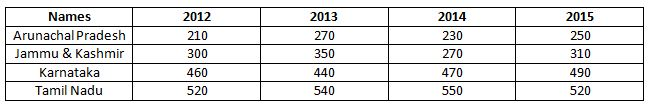Study the table carefully and answer the questions that follow: Question 18: If we consider each of the numbers for Tamil Nadu as an observation, what is the “mode” of the observations over the four years? a) 532 b) 530 c) 527 d) 520 Instructions The total usage of pesticides and fertilisers in the state of Kerala from 2001 to 2004 is as shown in the diagram below.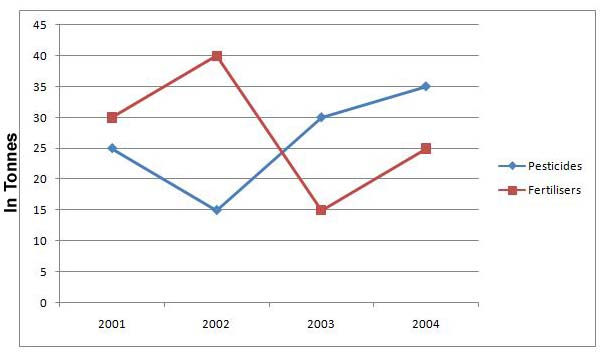Study the chart carefully and answer the questions that follow: Question 19: If the cost of pesticides is Rs. 2500 per tonne and cost of fertilisers is Rs. 3000 per tonne, on which of the following did the state of Kerala spend more money on, over all the years combined? a) Fertilizers b) Pesticides c) Same on both d) Can’t be determined Instructions The below chart gives the distribution of traffic caused by various types of vehicles. Using the information given in the chart, answer the following questions.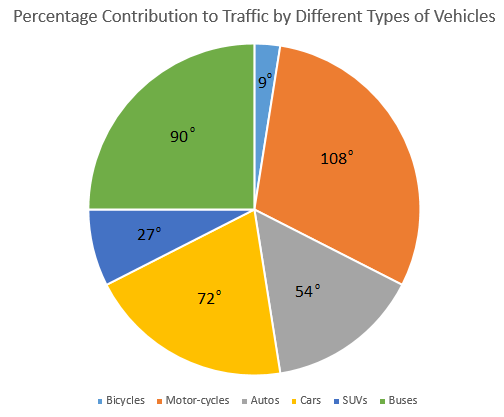Question 20: What percentage of the total traffic is due to buses and SUVs? a) 27.5% b) 30% c) 32.5% d) 35% Instructions The following table shows the sectoral distribution of production of Fabrics in India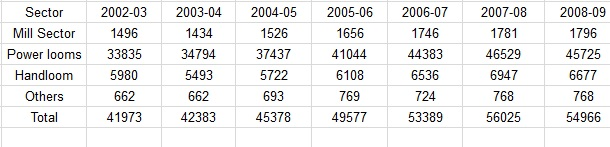Question 21: Read the statement below and answer the question that follow: Statement I:The contribution of mill sector to the total production of Fabrics in India does not exceed 4%. Statement II.The ratio of the production by mill sector to that of Handloom sector in 2004-05 and 2006-07 are almost same. a) Only statement I is true b) Only statement II is true c) Both the statement I and II are true d) Both the statement I and II are false Question 22: Find 70% of 10% of 500% of 20 ? a) 14 b) 7 c) 21 d) 28 Question 23: 20% of 30% of 400% of 50% of 60 = ? a) 8.4 b) 7.2 c) 6.8 d) 8.2 Question 24: If circumference of a circle is increased by 10%, the area of the circle will increase by a) 5% b) 10% c) 20% d) 21% Question 25: In a school 10% of boys is equal to$ \frac {1}{4} $th of the number of girls. What is the ratio of the boys and girls in that school ? a) 3: 2 b) 5: 2 c) 2: 1 d) 4: 3 Question 26: Raghu deposits Rs. 2000 in a bank which gives him a compound interest at 10% p.a. The total amount he receives is Rs. 2662. What is the number of years he deposited the money? a) 3 b) 2 c) 4 d) 2.5 Question 27: In a store, the shopkeeper sells at a profit percentage of 240%. Due to inflation, the cost price is increased by 30% while the selling price remains the same. In the new scenario, what percentage of the selling price is the profit ? a) 63.76% b) 62.76% c) 61.76% d) 60.76% Question 28: Amit invests Rs. 20,000 in two banks. He invested half of the sum in a bank that pays compound interest and half in a bank that pays simple interest. The interest rate in both the banks is 10% p.a. How much interest will he make at the end of 2 years? a) Rs. 2,000 b) Rs. 2,100 c) Rs. 4,000 d) Rs. 4,100 Question 29: Find the net profit % if the mark up % is 30% and the discount% is 10%? a) 13% b) 21% c) 15% d) 17% Question 30: By selling 80 articles, the shopkeeper gains the CP of 20 articles. Find the gain percentage? a) 25% b) 20% c) 30% d) 35% Question 31: Find the number of prime factors of 12400. a) 4 b) 3 c) 5 d) 6 Question 32: How many positive factors of 24 are there? a) 3 b) 4 c) 6 d) 8 Question 33: What is the value of$\frac{\text{0.03 x 4.2 + 7.9 x 0.75 + 0.4 x 0.15} }{\text{3.2 x 0.06 + 3.75 x 0.4 + 3.03 x 0.45}}$? a) 2 b) 3 c) 5 d) 7 Question 34: Sum of four consecutive odd numbers is 208. Find the largest number. a) 55 b) 57 c) 59 d) 61 Question 35: A certain sum will amount to 12,100 in 2 years at 10% per annum of compound interest, interest being compounded annually. The sum is a) 8000 b) 6000 c) 12000 d) 10000 Question 36: Two numbers ‘a’ and ‘b’ are such that the sum of 8% of a and 4% of b is equal to three fourth of the sum of 9% of a and 8% of b. Find the ratio of a and b? a) 4:3 b) 8:5 c) 7:4 d) 2:1 Question 37: If the sum of the squares of two positive numbers is 205 and difference of these numbers is 7. The biggest number is a) 16 b) 12 c) 13 d) 17 Instructions In a group of 80 people, there are doctors, politicians and cricketers. Sixteen people are only doctors, ten people are only cricketers and 12 people are only politicians. Six people are both doctors and cricketers but not politicians and four people are doctors, cricketers and politicians. The total number of cricketers in the group is 28. Each of the persons in the group has at least one profession. Question 38: How many politicians are also doctors? a) 4 b) 24 c) 28 d) 32 Question 39: If A and B together can complete a piece of work in 18 days, B and C together can complete the same piece of work in 36 days and C and A together can complete the same piece of work in 24 days, then in how many days can A, B and C together can complete the same piece of work? a) 8 b) 12 c) 14 d) 16 Question 40: 2 runners start running from the opposite sides of a track AB. The speed of the runner who starts running from A towards B is 30 kmph and the speed of the runner who starts running from B to A is 40 kmph. If the length of the track is 200 m, at what distance from A will the two runners meet? a) 400/7 m b) 800/7 m c) 500/ 7 m d) 600/7 m Answers & Solutions: 1) Answer (A)$(a+b+c)(a+2b-c)$=$a^2+2ab-ac+ab+2b^2-bc+ac+2bc-c^2$=$a^2+2b^2-c^2+3ab+bc$So the answer is option A. 2) Answer (C) For any given number, that can be represented as$ A^{x} \times B ^ {y} $, etc The number of factors is denoted by (x+1) x ( y+1), etc 360 =$ 2 ^ {3} \times 3 ^ {2} \times 5^ {1}$So the number of factors = (3 +1) x (2+ 1) (1+ 1) = 4x3x2 = 24 For 240, it is$ 2 ^ {4} \times 3 ^ {1} \times 5^ {1}$Number of factors = 5 x 2 x 2 = 20 only 3) Answer (B) Let the number be x. => (9+x)(83+x) = (23+x)(41+x) =>$747 + 92x + x^2 = 943 + 64x + x^2$=> 28x = 196 => x = 7 4) Answer (C) After the students left, there will be 32 students with the same average height. 4 ft and 2 inches Total height = 32 x 4.17 = 133.44 Total height of 6 students is 33 ft and 4 inches. = 33.33 New total height = 133.44 + 33.33 = 166.77 New average height = 166.77/ 38 = 4 feet 4 inches 5) Answer (C) The average age increases with age. So, after 10 years, the average age is 20 + 10 = 30 years. 6) Answer (A) Incomes of Ravi, Rahul and Ramesh are in the ratio 3:4:6. => Let the incomes of Ravi, Rahul and Ramesh be 3x, 4x and 6x respectively. Incomes of Ravi, Rahul and Ramesh are increased by 20%, 40% and 60% respectively. => New income of Ravi = 3x * 1.2 = 3.6x => New income of Rahul = 4x * 1.4 = 5.6x => New income of Ramesh = 6x * 1.6x = 9.6x Ratio of new incomes of Ravi, Rahul and Ramesh = 3.6x : 5.6x : 9.6x = 9 : 14 : 24 7) Answer (D) 4/5th of 100=80 no of remaining pages=100 – 80=20 8) Answer (B) 9) Answer (D) Since the ratio of sides is given as 4:5:6, let the side of the triangle be 4y,5y and 6y. The perimeter is given as 90.Thus, 90=4y + 5y + 6y=15y => y=6. Thus, the sides are 24,30,36. Area of the triangle by Heron’s formula=√s(s-a)(s-b)(s-c)=357.14 , where s is the semiperimeter and a,b,c are the sides of the triangle. Since no options satisfy the answer, option D none of these is correct. 10) Answer (A) From the information given, we can draw the family graph as shown.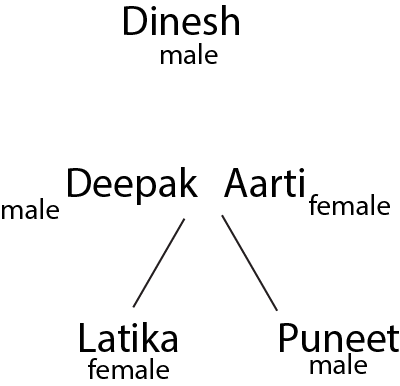We know that Dinesh is Latika’s grandfather but we cannot say if he is Deepak’s father or Aarti’s. Hence, we cannot say option B. Dinesh is Puneet’s grandfather. Option A is true. 11) Answer (C) Mother’s brother will be his Maternal Uncle and his wife will be her maternal aunt. Hence, answer will be c 12) Answer (A) There are 8 members and 3 couples in the family. There are 2 grand children in the family => If these 2 children are considered to b of generation 0, then the members of the family in generations -1 and -2 are all married because there have to 3 couples in the family. E is one of the grand children of F => F is a female belonging to generation -2. As E is a nephew of someone in the family, E is a male belonging to generation 0. D is married to H => These two members cannot belong to generation -2. They have to be in generation -1. D is a male and H is a female. H is the aunt of B => B belongs to generation 0. B’s mother is A => A belongs to generation -1 and is a female C is the oldest member of the family => C is a male member who is married to F and belongs to generation -2. G is the only person left and he has to be from generation -1 as he has children => G is married to A and both E and B are their children. G has a son and a daughter. E is a male => B is a female of generation 0. C has two sons in generation -1 => G and D who are the only male members in generation -1 are the sons of C. D has 0 children.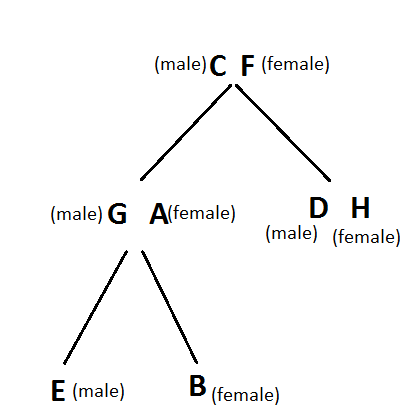13) Answer (D) B is the brother of A. Q is the brother of B. P is the brother of Q and R is the brother of P. So, all the 5 people are brothers. There is no uncle of A among the other 4. Option d) is the correct answer. 14) Answer (A) Abhijeet is the brother of Soumya. Soumya’s son is Arya. So, Abhijeet is Arya’s maternal uncle. 15) Answer (B) 1994 has 365 days average days per month =$\frac{365}{12} = 30.4166$So the answer is option B. 16) Answer (B) For each non-leap year, 1st jan will shift to one day of the week earlier. Hence, 1st jan 2015 would be on Thursday. As 1st Jan falls before 29th February, for leap years we need to deduct 2 days. From 2002 to 2016, there are 11 non-leap year and 3 leap years. Hence, we need to deduct = 11*-1+3*-2= -17. Hence, we need to count 17 days earlier, which is the same as 2 weeks and 3 days. Hence, Friday – 3 days of the week = Tuesday. Hence, 1st January 2002 was a Tuesday. 17) Answer (D) Every year the day increases by one and in every leap year the day increases by 2. So, if August 1 2007 was a Saturday, August 1 2008 is a Monday (Since 2008 is a leap year). August 1 2009 is a Tuesday. August 1 2010 is a Wednesday and August 1 2011 is a Thursday. 18) Answer (D) The values for Tamil Nadu are: 520, 540, 550 and 520. Since 520 repeats twice (more times than any other value), it is the mode of the observations. 19) Answer (A) Total pesticides used = 25 + 15 + 30 + 35 = 105 tons Total fertilizers used = 30 + 40 + 15 + 25 = 110 tons Total cost of pesticides = 2500 * 105 = 262,500 Total cost of fertilizers = 3000 * 110 = 330,000 So, the cost of fertilizers is more 20) Answer (C) Angle made by SUVs = 27 degrees Angle made by Buses = 90 degrees Total angle made by buses and SUVs = 117 degrees. Total angle made by whole traffic = 360 degrees => % of traffic due to buses and SUVs =$\frac{117}{360}*100$= 32.5% 21) Answer (C) percentage of mill sector to that of fabrics from 2002-03 to 2008-09 is given below: 3.56, 3.38 ,3.36, 3.32, 3.27, 3.17, 3.26 thus, in no year the % is greater than 4.∴ statement 1 is correct. The ratio of mill sector to handloom in 2004-05 and 2005-06 are 26.67% and 27.1% respectively.∴ Statement 2 is also correct.Thus, option C is the right choice. 22) Answer (B) 70% of 10% of 500% of 20 = (0.7)(0.1)(5)(20) = (0.7)((0.1)(100) = (0.7)(10) = 7 So the answer is option B. 23) Answer (B)$(0.2)(0.3)(4)(0.5)(60) = (0.2)(0.3)(120) = (0.06)(120) = 7.2$So the answer is option B. 24) Answer (D) Circumference of a circle is 2$ \pi$r So, if the circumference increases by 10%, the radius also increases by 10% area of the circle =$ \pi r ^ {2}$If radius becomes 1.1r, area increases by$1.1 ^ {2}$= 1.21 25) Answer (B) 10% of the boys = 1/4 of the number of girls. So b/10 = g/4 b/g = 10/4 = 5/2 26) Answer (A) Total amount =$P(1+\frac{r}{100})^n2662 = 2000(1+\frac{10}{100})^n2662 = 2000(11/10)^n1.331 = (11/10)^n(11/10)^3 = (11/10)^n n = 3$So the answer is option B. 27) Answer (C) Let CP = 100 Profit = 240% of 100 = 240 SP = CP+profit = 100+240 = 340 If CP is increased by 30%, New CP = 130 New SP = Unchanged = 340 Profit = 340 – 130 = 210/- Answer =$\frac{profit}{SP}\times 100 = \frac{210}{340}\times 100 = 61.76$% So the answer is option C. 28) Answer (D) The principal in the bank with simple interest is Rs. 20,000/2 = Rs. 10,000 Interest earned = 10,000 * 2 * 10% = Rs. 2,000 The principal in the bank with compound interest is Rs. 20,000/2 = Rs. 10,000 Interest earned =$10,000 * 1.1^2$– 10,000 = Rs. 2,100 Total interest earned = 2,000 + 2,100 = Rs. 4,100 29) Answer (D) Let the CP = 100 Mark Up = 30% MP = 130 Disount = 10% SP = 90% of 130 = 117 Gain% = (117-100)/100 = 0.17 = 17% 30) Answer (A) SP of 80 articles = CP of 80 articles+CP of 20 articles SP of 80 articles = CP of 100 articles SP/CP = 100/80 = 1.25 Gain% = (1.25-1)*100 = 25% 31) Answer (B) We can factorize the number in form of prime factors.$12400 = 2^4*5^2*31^1$Therefore, the number of prime factors = 3. Hence, option B is the correct answer. 32) Answer (D) Prime factorization of$24=(2)^3\times(3)^1$=> umber of positive factors =$(3+1)\times(1+1)$=$4\times2=8$=> Ans – (D) 33) Answer (A)$\frac{\text{0.03 x 4.2 + 7.9 x 0.75 + 0.4 x 0.15} }{\text{3.2 x 0.06 + 3.75 x 0.4 + 3.03 x 0.45}}$=$\frac{\text{0.126 + 5.925 + 0.06}}{\text{0.192 + 1.5+ 1.3635}}$=$\frac{6.1110}{3.0555}$= 2 Hence, the option A is correct. 34) Answer (A) Let the four consecutive odd numbers be$(x-3),(x-1),(x+1)$and$(x+3)$Sum =$(x-3)+(x-1)+(x+1)+(x+3)=208$=>$4x=208$=>$x=\frac{208}{4}=52\therefore$Largest number =$52+3=55$=> Ans – (A) 35) Answer (D) Let the sum =$x$rate = 10% and time = 2 years Amount after compound interest =$x (1 + \frac{10}{100})^{2}$= 12100 =>$\frac{121x}{100}$= 12100 =>$x$= 10,000 36) Answer (B) We have been given that sum of 8% of a and 4% of b is equal to three fourth of the sum of 9% of a and 8% of b So we have$\frac{8a}{100} + \frac{4b}{100} = \frac{3}{4}(\frac{9a}{100} + \frac{8b}{100})$=>$ 8a + 4b = \frac{3}{4}(9a + 8b) 32a + 16b = 27a + 24b$=> 5a = 8b =>$\frac{a}{b} = \frac{8}{5}$37) Answer (C) Let x and y be the biggest and smallest numbers respectively$x^2 + y^2 = 205$x-y = 7 ==>$(x-y)^2 = 7^2$==>$x^2 + y^2 -2*x*y = 49$==>$205 – 2*x*y = 49$==>$2*x*y = 205-49$==>$x*y = 78$==> x = 78/y ==> 78/y -y = 7 ==>$78 – y^2 = 7y$On solving the above equation y = 6 and x = 13 Therefore, the biggest number is 13. 38) Answer (C) Mark the information given in a venn-diagram.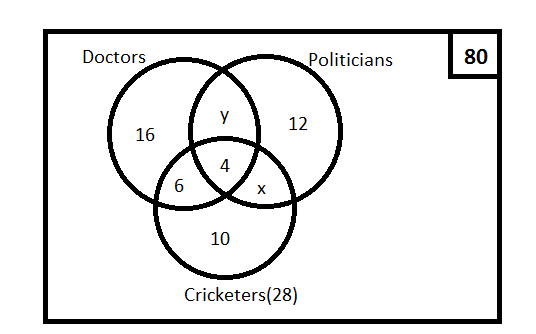Total number of cricketers = 28 We can the find the number of people who are both cricketers and politicians with the given information. 10 + 6 + x + 4 = 28 => x = 8 => Number of people who are both cricketers and politicians is 8. Similarly, we can find the number of people who are both doctors and politicians. 16 + 12 + 10 + y + 6 + 8 + 4 = 80 => y = 24 => Number of people who are both doctors and politicians is 24. Number of politicians who are also doctors is 24 + 4 = 28. 39) Answer (D)$\frac{1}{a} + \frac{1}{b}$=$\frac{1}{18}\frac{1}{a} + \frac{1}{c}$=$\frac{1}{24}\frac{1}{b} + \frac{1}{c}$=$\frac{1}{36}$=> 2($\frac{1}{a} + \frac{1}{b} + \frac{1}{c}$) =$\frac{1}{18}$+$\frac{1}{24}$+$\frac{1}{36}$=$\frac{1}{8}$=> ($\frac{1}{a} + \frac{1}{b} + \frac{1}{c}$) =$\frac{1}{16}\$
=> It takes 16 days for A, B and C together to complete the work.